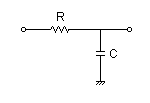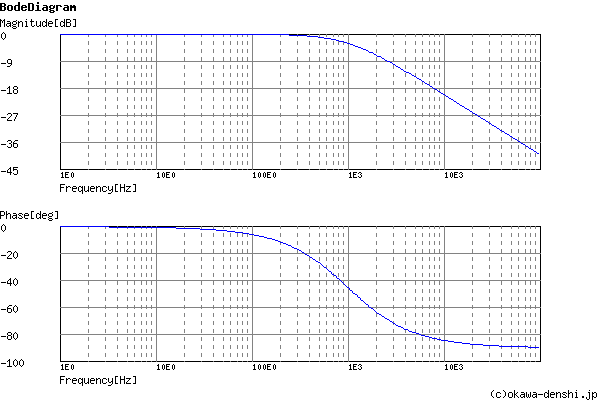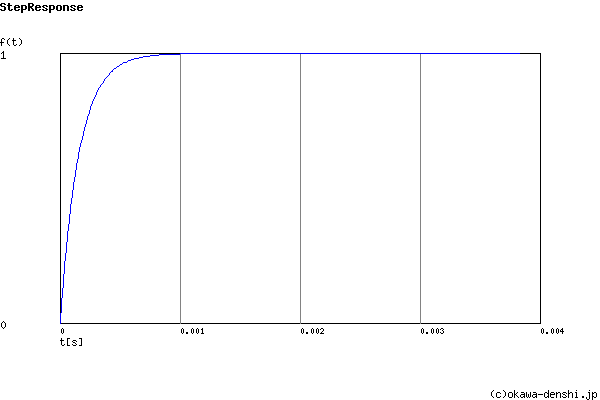# (Sample)RC Low-pass Filter Design Tool - Result -

Calculated the Transfer Function for the RC Low-pass filter, displayed on a graph, showing Bode diagram, Nyquist diagram, Impulse response and Step response.

## CR Filter

 Vin(s)→→Vout(s)
(Sample)Transfer Function:
 G(s)= 6250 s+6250

R = 1.6kΩ
C = 0.1uF

fc = 1000[Hz]

### Rise/Fall time of step response

fc = Hz

tr = [sec]

#### Pole(s)

p = -994.718394324[Hz]
|p|= 994.718394324[Hz]

#### Final value of the step response (on the condition that the system converged when t goes to infinity)

g(∞) = 1

 fc=Hz C=F (Omitted C=1, 0.1, 0.01…) p:pico, n:nano, u:micro, k:kilo, M:mega Select Resistor Sequence: E24 E12 E6 E96

### Frequency analysis

Bode diagram
Phase  Group delay
Nyquist diagram
Pole, zero
Phase margin
Oscillation analysis
Analysis on frequency range:
f1=∼f2=[Hz] (optional)

### Transient analysis

Step response
Impulse response
Overshoot
Final value of the step response
Analysis on time range:
0∼[sec] (optional)

# Frequency analysis# Transient analysis